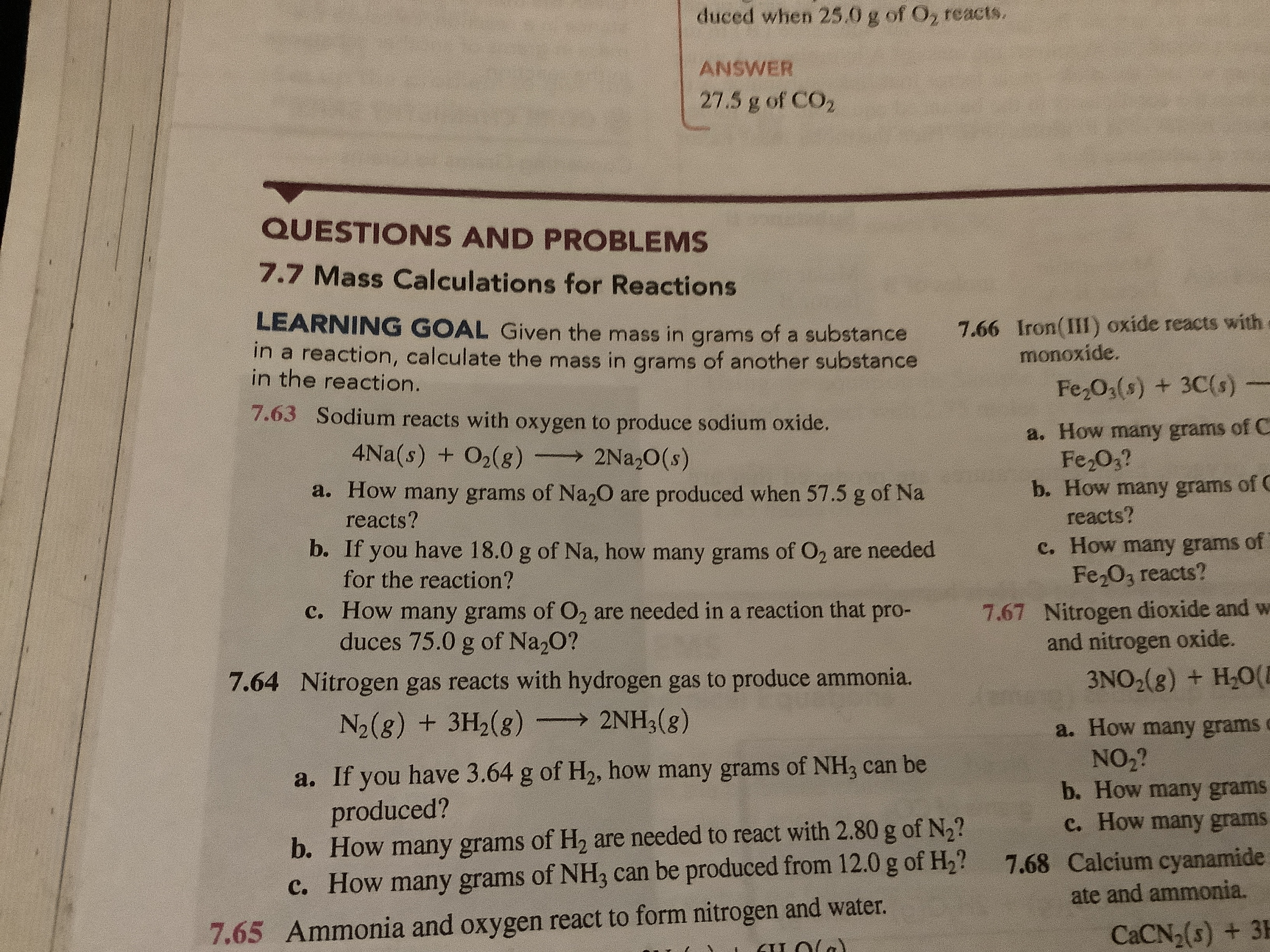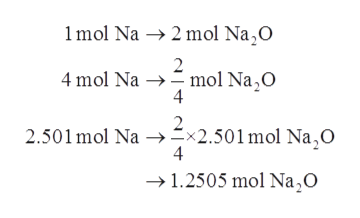# duced when 25.0 g of O2 reacts.ANSWER27.5 g of CO2QUESTIONS AND PROBLEMS7.7 Mass Calculations for Reactions7.66 Iron(III) oxide reacts withmonoxide.LEARNING GOAL Given the mass in grams of a substancein a reaction, calculate the mass in grams of another substancein the reaction.Fe2O3(s) + 3C(s)7.63 Sodium reacts with oxygen to produce sodium oxide.a. How many grams of CFe 02?b. How many grams of Creacts?c. How many grams ofFe2O3 reacts?Nitrogen dioxide and wand nitrogen oxide.4Na(s) + O2(8)2Na,O(s)a. How many grams of Na20 are produced when 57.5 g of Nareacts?b. Ifhave 18.0 g of Na, how many grams of O2 are neededyoufor the reaction?c. How many grams of O2 are needed ina reaction thatduces 75.0 g of Na20?pro-7.677.64 Nitrogen gas reacts with hydrogen gas to produce ammonia.2NH3(8)3NO2(8) + H20(EN2(g) + 3H2(8)a. How many gramsNO2?b. How many gramsc. How many gramsa. If you have 3.64 g of H2, how many grams of NH3 can beproduced?b. How many grams of H2 are needed to react with 2.80 g of N2?c. How many grams of NH3 can be produced from 12.0 g of H2?7.68 Calcium cyanamideate and ammonia.Ammonia and oxygen react to form nitrogen and water.CaCN2(s) + 3H7.65

Question

Question 7.63help_outlineImage Transcriptioncloseduced when 25.0 g of O2 reacts. ANSWER 27.5 g of CO2 QUESTIONS AND PROBLEMS 7.7 Mass Calculations for Reactions 7.66 Iron(III) oxide reacts with monoxide. LEARNING GOAL Given the mass in grams of a substance in a reaction, calculate the mass in grams of another substance in the reaction. Fe2O3(s) + 3C(s) 7.63 Sodium reacts with oxygen to produce sodium oxide. a. How many grams of C Fe 02? b. How many grams of C reacts? c. How many grams of Fe2O3 reacts? Nitrogen dioxide and w and nitrogen oxide. 4Na(s) + O2(8) 2Na,O(s) a. How many grams of Na20 are produced when 57.5 g of Na reacts? b. If have 18.0 g of Na, how many grams of O2 are needed you for the reaction? c. How many grams of O2 are needed ina reaction that duces 75.0 g of Na20? pro- 7.67 7.64 Nitrogen gas reacts with hydrogen gas to produce ammonia. 2NH3(8) 3NO2(8) + H20(E N2(g) + 3H2(8) a. How many grams NO2? b. How many grams c. How many grams a. If you have 3.64 g of H2, how many grams of NH3 can be produced? b. How many grams of H2 are needed to react with 2.80 g of N2? c. How many grams of NH3 can be produced from 12.0 g of H2? 7.68 Calcium cyanamide ate and ammonia. Ammonia and oxygen react to form nitrogen and water. CaCN2(s) + 3H 7.65 fullscreen
check_circleExpert Solution
Step 1

Given information:

The chemical equation for reaction between solid sodium and oxygen gas is represented as follows:

Step 2

a. First, number of moles of sodium will be calculated using atomic weight of sodium as follows:

Step 3

After calculating moles of sodium moles of sodium oxide (Na2O) will be calculated u...help_outlineImage Transcriptionclose1mol Na 2 mol Na,O 2 4 mol Na mol Na,O 4 2.501mol Na x2.501 mol Na,0 4 1.2505 mol Na,O fullscreen

### Want to see the full answer?

See Solution

#### Want to see this answer and more?

Solutions are written by subject experts who are available 24/7. Questions are typically answered within 1 hour*

See Solution
*Response times may vary by subject and question
Tagged in

### Chemistry